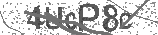﻿ Basic Trigonometry - Practice Quiz | Safe Videos for Kids
Welcome

# Basic Trigonometry - Practice Quiz

Thanks! Share it with your friends!

URL

You disliked this video. Thanks for the feedback!

Sorry, only registred users can create playlists.URLFind Related Videos  added
308 Views## Description

Learn basic trigonometry with this fun interactive math quiz. You can skip questions by clicking on the time stamps below. I also provided the solutions to every question.

Timeline
0:09 - Question #1 - Basic Trigonometry Quiz
0:37 - Question #2 - Basic Trigonometry Quiz
0:57 - Question #3 - Basic Trigonometry Quiz
1:17 - Question #4 - Basic Trigonometry Quiz
1:44 - Question #5 - Basic Trigonometry Quiz
2:15 - Answer #1 - Basic Trigonometry Quiz
2:45 - Answer #2 - Basic Trigonometry Quiz
3:03 - Answer #3 - Basic Trigonometry Quiz
3:16 - Answer #4 - Basic Trigonometry Quiz
3:31 - Answer #5 - Basic Trigonometry QuizBe the first to comment